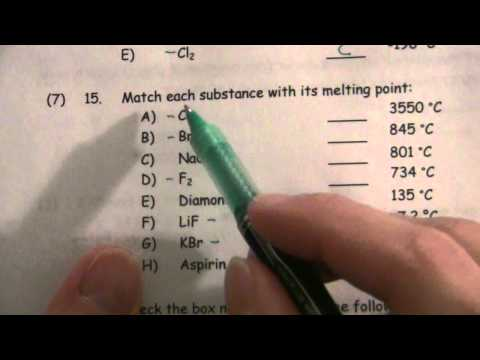### Main Difference

Boiling Point and Melting point are the terms related to the change of state of matter as both of them are the temperatures when intermolecular forces are weaken and with increase in kinetic energy molecules are set to change their state of matter. Melting point is the temperature when solid starts converting into liquid. For instance, the melting point of ice is 0°C or 273K, so at this temperature ice will start breaking down as a liquid. Boiling Point is the temperature when liquid starts getting transform into vapors form. For instance, at room temperature Boiling point of water is 100°C, at this temperature water molecules start evaporating as vapors.

### Comparison Chart

 Boiling Point Melting Point Definition The boiling point is the temperature at which the vapor pressure of a liquid is equal to the external pressure surrounding the liquid. The melting point is the temperature at which solid and liquid phases are in thermal equilibrium state. Physical Change Boiling point is the temperature when liquid starts getting transform into vapors form. Melting point is the temperature when solid starts converting into liquid. Pressure The boiling point doesn’t remain same it changes with the inference of the external pressure. The melting point has nothing to do with external pressure.

### What is Boiling Point?

The boiling point is the temperature at which the vapor pressure of a liquid is equal to the external pressure surrounding the liquid. Usually, this is a high temperature when intermolecular forces are weaken and molecules are all set to break the bond and to evaporate as vapor. External pressure is one of the most notable factor affecting the boiling process as higher the external pressure higher will be the boiling point, and lower the external pressure, lower the boiling point. The boiling point of water doesn’t remain same it changes with the inference of the external pressure. For example, at room temperature water has boiling point of 100°C, though at Mount Everest where pressure is about 34 kPa boiling pint of water is 71 °C.

### What is Melting Point?

The melting point is the temperature at which solid and liquid phases are in thermal equilibrium state. Melting point is generally the property of solids. It is the certain temperature when solids get transforming as liquids. As we know that in solids molecules are tightly held through the inter-molecular forces, so when it’s about the melting point the kinetic energy is quite high to free the molecules so they could change the state of matter. Many people deriving from it think that melting and freezing point of a substance are same, though it isn’t compulsory as in the case of agar, which melts at 85 0C but gets back in solid form at 31 0C to 40 0C.

### Boiling Point vs. Melting Point

• The boiling point is the temperature at which the vapor pressure of a liquid is equal to the external pressure surrounding the liquid, while the melting point is the temperature at which solid and liquid phases are in thermal equilibrium state.
• Melting point is the temperature when solid starts converting into liquid, whereas boiling point is the temperature when liquid starts getting transform into vapors form.
• The boiling point doesn’t remain same it changes with the inference of the external pressure, whereas melting point has nothing to do with external pressure.
• Melting and freezing point of a substance are not the same as in the case of agar, which melts at 85 0C but gets back in solid form at 31 0C to 40 0C.
• The boiling point of water doesn’t remain same it changes with the inference of the external pressure. For example, at room temperature water has boiling point of 100°C, though at Mount Everest where pressure is about 34 kPa boiling pint of water is 71 °C.